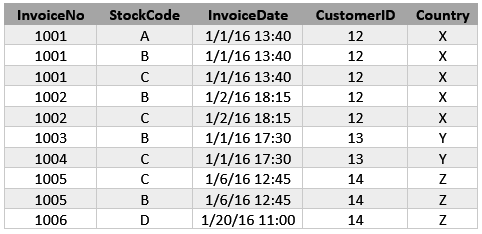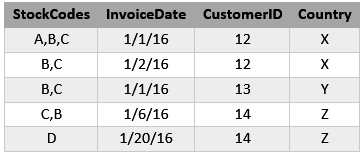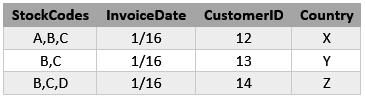# Fourth Approach: Sequential rules mining

In this last part, we want to extract sequential rules from the dataset. In the previous part, we extracted association rules which tell us if a customer buys product X then he/she will buy product Y. The sequence of purchased was not important. But here instead, we want extract rules similar to: “If a customer buys X then in the next purchase he/she will buy Y”. As you can see here the sequences are important. In other words, we want to extract association rules which happened during the specific period of time.

The below dataset is the transactional dataset. If we consider the time sequence as one day then the second table shows the aggregation of customer purchases based on one day period:Moreover, if we extend the time span to one month then the table of customers and products will be as follow:But on the last dataset, we can not find any sequence because all of the customers have just one sequence and if you run sequential pattern mining algorithm on this you won’t get any result. Again, we have to first construct our dataset so that we can apply our desire algorithm on it.

#Load the data
dt1$InvoiceDate <- as.POSIXct(dt1$InvoiceDate, format = "%m/%d/%Y %H:%M")

We created a very nice and sophisticated function using which one can combine all transactions based on specific IDs for the desired time span and then use it for cSPADE algorithm in order to extract sequential pattern rules. This function accepts data1 which is the dataset, datevarpos which indicates the position of column for the date variable, idvarpos indicates the position of ID variable (which here is customer ID), transvarpos which indicates the column position of entity in the transaction (which here are products) and finally ztime which indicates the time for the aggregation of transaction based on day. For example, if you set the ztime as 1 it will combine all of the products which are purchased by the customer on the same day and if you put 30 it will combine all of the products which are purchased by customer during 90 days (starting from the first date). If you want to see the progression in the algorithm you might uncomment “#print(paste0..” this line.

library(dplyr)
library(arulesSequences)
srfun1 <- function(data1,datevarpos=3,idvarpos=4,transvarpos=2,ztime){
#Convert variables to desired name
names(data1)[datevarpos] <- "Datevar"
names(data1)[idvarpos] <- "VisitId"
names(data1)[transvarpos] <- "Code"
#Aggregate data based on Id variables
df <- data1 %>%
arrange(Code) %>%
unique() %>%
group_by(VisitId,Datevar) %>%
summarise(cart=paste(Code,collapse=";")) %>%
ungroup()
#Extract the unique visitIds
un1 <- unique(df$VisitId) z=c() #For loop for extracting the pattern based on ztime for (k in 1:length(un1)){ y=c() j=1 ln1=length(unique(df[df$VisitId==un1[k],]['Datevar'])[])-1
if (ln1<1){
z[k]=list("1")
}else{
for(i in 1:ln1){
if (unique(df[df$VisitId==un1[k],]['Datevar'])[][i+1] <= unique(df[df$VisitId==un1[k],]['Datevar'])[][i]+ztime*86400){
y[i]=paste0(j)
}
if(unique(df[df$VisitId==un1[k],]['Datevar'])[][i+1] > unique(df[df$VisitId==un1[k],]['Datevar'])[][i]+ztime*86400){
j=j+1
y[i]=paste0(j)
}
}
z[k] <- list(append(1,y))
}
#print(paste0("Extracting ",k," from ",length(un1)))
}
#Add concatenated data to the original data and cosruct new dataset
df$newz <- unlist(z) df$visn1 <- paste0(df$VisitId,"_",df$newz)
df3 <- df %>%
arrange(cart) %>%
unique() %>%
group_by(visn1) %>%
summarise(cart1=paste(cart,collapse=";")) %>%
ungroup()
df3$visid <- gsub(pattern ="_(.*)" ,replacement ="" ,x = df3$visn1)
df3$seq1 <- gsub(pattern ="(.*)_" ,replacement ="" ,x = df3$visn1)
for (i in 1:nrow(df3)){
df3$cart2[i] <- lapply(list(unique(sort(as.numeric(unlist(strsplit(df3$cart1[i],";")))))),paste, collapse = ";")
}
print("Preparing the data. You have to wait depending on the size of the dataset.")
#Construct dataset so that we can use in cSPADE
df3$cart2 <- gsub(";"," ",df3$cart2)
df3$SIZE <- count.fields(textConnection(df3$cart2),sep = " ")
dt.out <- as.data.frame(cbind(df3$visid,df3$seq1,df3$SIZE,df3$cart2))
dt.out$V2 <- as.numeric(as.character(dt.out$V2))
dt.out <- dt.out[order(dt.out$V1,dt.out$V2),]
write.table(dt.out, "mytxtout.txt", sep=" ", row.names = FALSE, col.names = FALSE, quote = FALSE)
}

The output of this function would be transactions containing the items, SequencID, evenID, and SIZE. For information about the sequential rules mining in R you can take a look at this weblog. The trnew.dt contains the data frame of sequential transactions.

#Constructing transctions
trnew <- srfun1(dt1,datevarpos =3 ,idvarpos =4 ,transvarpos =2 ,1)
## Warning: Unknown column 'cart2'
##  "Preparing the data. You have to wait depending on the size of the dataset."
inspect(head(trnew))
##     items                                             sequenceID eventID
##  {22195,22725,22729}                               12347      1
##  {21035,21154,22725,22729,47559}                   12347      2
##  {21791,21975,22195,22432,22550,23076}             12347      3
##  {22725,23171,23172,23175,51014}                   12347      4
##  {21578,21791,21975,22992,23175,23297,23308,47559} 12347      5
##  {21791,22195,22561,22621,22725,22992,23308,47559} 12347      6
##     SIZE
##  3
##  5
##  6
##  5
##  8
##  8
trnew.dt <- as(trnew,"data.frame")

Now we can apply cSPADE algorithm on the dataset. For CSAPDE algoritm you might set some lags so that you can extract rules from sequence of transactions with the lag. We set the minimum support of rules to 4.5%. Following codes demonstrate this procedure:

#Run CSpade algorithm (support here is 0.01 which can be changed)
sc.rul <-cspade(trnew,parameter = list(support = 0.045))
#Convert extracted sequential rules to data frame
scrul.dt <- as(sc.rul,"data.frame")
scrul.dt$sequence <- gsub("df3\\$cart2\\=|<|>","",scrul.dt$sequence) Some of these rules have just one sequence so the following code will filter out those rules and keep rules with more than one sequnce. All the rules will be store in “scrul.dt1” which is a dataframe: #Filter rules with more than one sequence scrul.dt1 <- scrul.dt[count.fields(textConnection(scrul.dt$sequence),sep = ",")>1,]
scrul.dt1[47,]
##                sequence    support
## 277 {21239,21240,20675} 0.04591837

For example, rule 47 says that “If a customer’s first purchase is 21239, his second purchase would be 21240, followed by his third purchase of 20675” wich is frequent for 5% of customers. As we set ztime=1 it means that each of unique sequences happened on the same date. By changing “SPparameter” part and specifying mingap or maxgap you can add some lag time to extracted rules. You can download the code from this link and data here